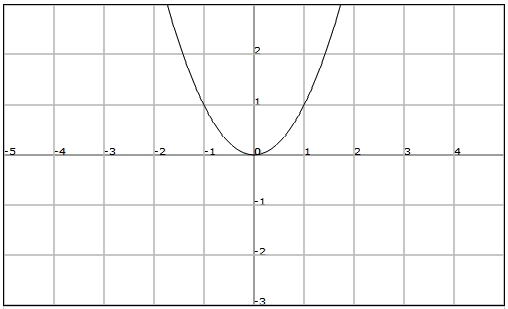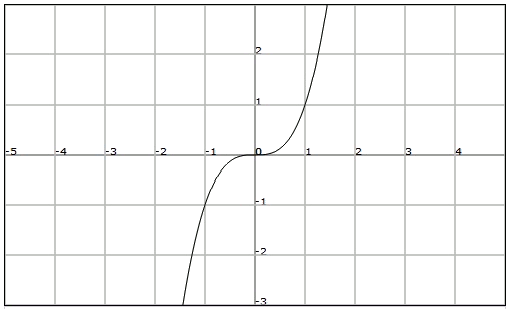# Even and odd functions

Let's consider the graph of the following function $$f(x)=x^2$$:We observe that any number $$x$$ and its opposite $$-x$$ have the same image. In this case we say that the function is a pair.

A function $$f$$ is a pair if for any $$x$$ in the domain we have: $$f(x)=f(-x)$$$Note that even functions are symmetrical with regard to the vertical axis. Let's consider now the function $$f(x)=x^3$$:We know that any number $$x$$ and its inverse $$-x$$ have inverse images. In this case we say that the function $$f$$ is odd. A function $$f$$ is odd if for any $$x$$ in the domain we have:$$f(x)=-f(-x)$$$

Given following functions, decide which of them are even or odd: $$\displaystyle f(x)=\frac{1}{x} \mbox{ and } g(x)=x^2-2$$$Let's verify if the functions are even: $$f(x)=f(-1) \Longleftrightarrow \displaystyle \frac{1}{x}=\frac{1}{-x} \Longleftrightarrow 1= -1 !! \\ g(x)=g(-x) \Longleftrightarrow x^2-2=(-x)^2-2=x^2-2 \mbox{ OK }$$$

Let's verify if the function $$f$$ is odd ($$g$$ will not be odd since it is an even function): $$\displaystyle f(x)=-f(-x) \Longleftrightarrow \frac{1}{x}=-\frac{1}{-x}=\frac{1}{x} \mbox{ OK }$$\$

Therefore the function $$f$$ is odd, and the function $$g$$ is a pair.